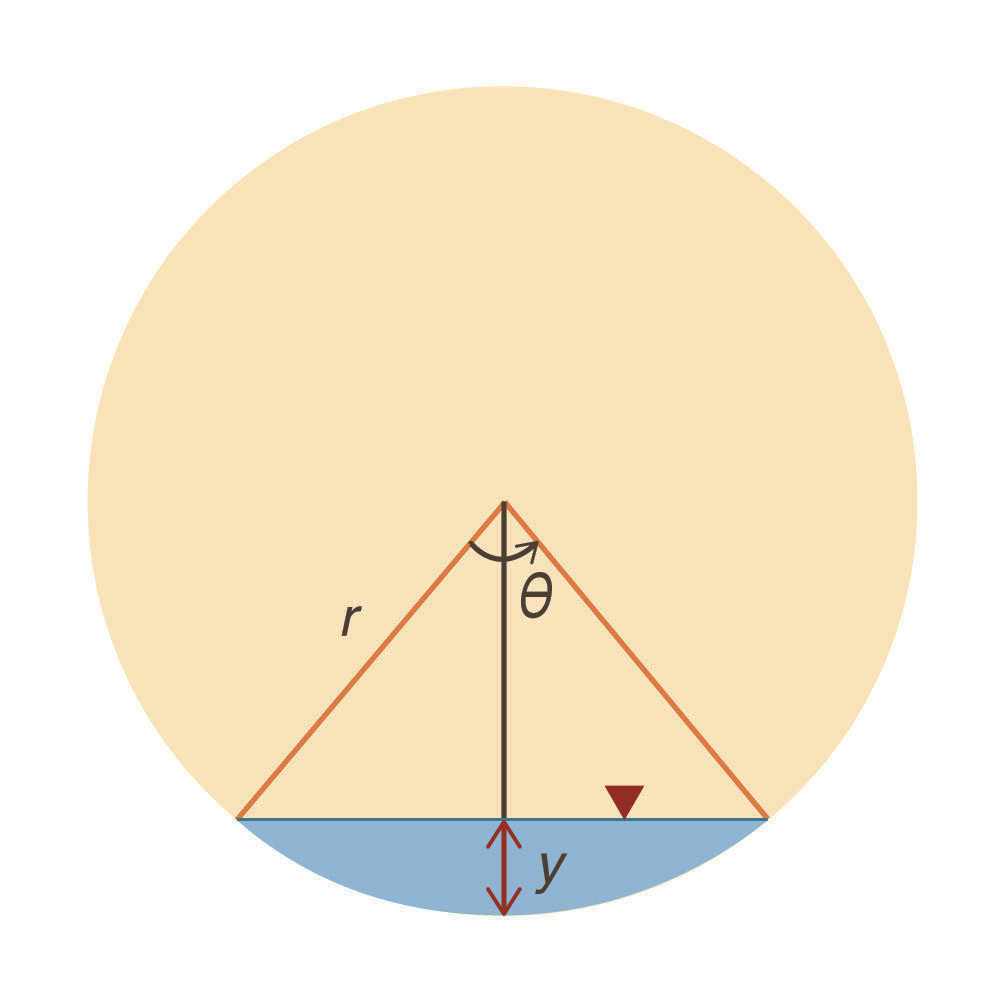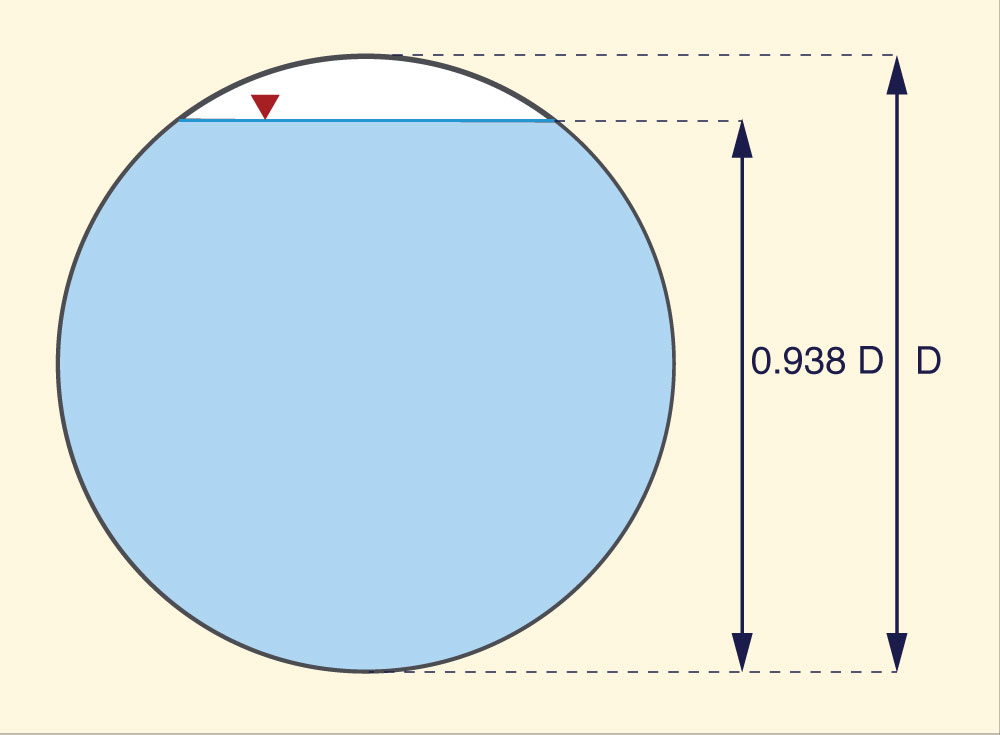What is the relative depthto achieve maximum dischargein a circular culvert? Tamina Igartua and Victor M. Ponce19 September 2015

 Abstract. Using differential calculus, the relative depth y/D required to achieve maximum discharge in a circular culvert is derived. This value is shown to be y/D = 0.938. The reason for this hydraulic behavior is that when the flow depth increases beyond the value y = 0.938 D, the wetted perimeter begins to grow faster than the flow area, causing the flow rate to begin to decrease.

1.  PROBLEM STATEMENT

The maximum discharge in a circular culvert occurs, not when the culvert is full, but when the culvert is nearly full. The reason for this is that beyond a certain relative depth y/D, where y is the flow depth and D is the culvert diameter, a further increase in depth will make the wetted perimeter grow faster than the flow area, triggering a decrease in discharge.

In this article, the relative depth y/D which corresponds to maximum discharge in circular culvert flow is calculated.

2.  DERIVATION

According to the Manning equation, the discharge in SI units is (Ponce, 2014):

 1 Q  =  ____ A R 2/3 S 1/2              n (1)

in which Q = discharge, A = flow area, R = hydraulic radius, S = bottom slope, and n = Manning's n. Since R = A/P, it follows that:

 1 Q  =  ____ A 5/3 P - 2/3 S 1/2              n (2)

In terms of r and θ, the flow area and wetted perimeter are, respectively (Fig. 1):

 r 2 A  =  ____   ( θ - sin θ )              2 (3)

 P  =  r θ (4)Fig. 1  Definition sketch.

Therefore:

 dA          r 2 _____  =  ____   ( 1 - cos θ )   dθ           2 (5)

 dP            _____  =  r       dθ (6)

By geometry, the relative depth is (Fig. 1):

 y /D  =  (1/2) [ 1 - cos (θ/2) ] (7)

Following differential calculus, the maximum discharge occurs when dQ/dθ = 0. Using Eq. 2, for a constant slope and Manning friction, this condition implies that:

 d        ____  ( A 5/3 P - 2/3 )  =  0  dθ (8)

Operating on the derivatives:

 5                        dA            2                         dP   ____  A 2/3 P -2/3  _____   -   ____  A 5/3 P - 5/3 _____  =  0    3                        dθ            3                         dθ (9)

Simplifying:

 dA                    dP    5 P   _____   -   2 A   _____  =  0            dθ                    dθ (10)

Replacing Equations 3 to 6 into Equation 10 and simplifying:

 5 θ ( 1 - cos θ )  -  2 ( θ - sin θ )  =  0 (11)

Simplifying Eq. 11:

 3θ  -  5θ cos θ  +  2 sin θ = 0 (12)

Solving for θ in Eq.10: θ = 302° 25' 51.96". This angle is used in Eq. 7 to solve for relative depth, to give y/D  =  0.938 (Fig. 2).Fig. 2   Relative depth for maximum discharge in a circular culvert..

3.  SUMMARY

The relative depth y/D required to achieve maximum discharge in a circular culvert has been derived using differential calculus. This value is shown to be: y/D = 0.938. The reason for this hydraulic behavior is that when the flow depth increases beyond the value y = 0.938 D, the wetted perimeter begins to grow faster than the flow area, causing the flow rate to decrease accordingly (Eq. 2).

REFERENCE

Ponce, V. M. 2014. Fundamentals of open-channel hydraulics. Online text.

 201223